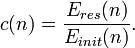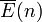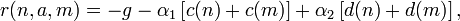# QELAR

Jump to: navigation, search

# Overview

QELAR is a Q-learning-based energy-efficient and lifetime-aware routing protocol. It is designed to address various issues related to underwater acoustic sensor networks (UW-ASNs). By learning the environment and evaluating an action-value function (Q-value), which gives the expected reward of taking an action in a given state, the distributed learning agent is able to make a decision automatically.

We find that Q-learning is very suitable in UW-ASNs in the following ways:

• Low Overhead. Nodes only keep the routing information of their direct neighbor nodes which is a small subset of the network. The routing information is updated by one-hop broadcasts rather than flooding.
• Dynamic Network Topology. Topology changes happen frequently in the harsh underwater environment. Without GPS available underwater, QELAR learns from the network environment and allows a fast adaptation to the current network topology.
• Load Balance. QELAR takes node energy into consideration in Q-learning, so that alternative paths can be chosen to use network nodes in a fair manner, in order to avoid 'hot spots' in the network.
• General Framework. Q-learning is a framework that can be easily extended. We can easily integrate other factors such as end-to-end delay and node density for extension and can balance all the factors according to our need by tuning the parameters in the reward function.

# Design

## Q-learning

Q-learning is one type of Reinforcement Learning algorithms, by which a system can learn to achieve a goal in control problems based on its experience. An agent in RL chooses actions according to the current state of a system and the reinforcement it receives from the environment. Most RL algorithms are based on estimating value functions, functions of states (or of state-action pairs), which evaluate how good it is for the agent to be in a given state (or how good it is to perform an action in a given state).

We denote the value of taking an action a in a state s as Q(s,a), and the direct reward of taking such an action as r(s,a). The optimal Q(s,a) can be approximated by the following iteration:$Q(s,a)\leftarrow(1-\alpha)Q(s,a)+\alpha\left[r(s,a)+\gamma\max_aQ(s',a)\right],$


where s' is the next state, α and γ is the learning rate and future discount, respectively.

## Reward Function

The reward function in Q-learning determines the behavior of the learning agent. In QELAR, we consider the following three rewards:

• The penalty of forwarding a packet. Forwarding a packet consumes energy, occupies channel bandwidth, and contributes to the delay. Therefore forwarding a packet should always receive a negative reward, which is g.
• Residual energy. Lower reward should be given if the residual energy of either the sender or the receiver is low. Therefore, forwarding to a packet to a node with low residual energy can be avoided. The reward related to residual energy of Node n is defined as$c(n)=\frac{E_{res}(n)}{E_{init}(n)}.$

• Energy distribution. Energy distribution should also be considered to ensure that the energy is distributed evenly among a group of sensor nodes, which includes the node n that holds a packet and all its direct neighbors in the transmission range. It is defined as$d(n)=\frac{2}{\pi}\arctan(E_{res}(n)-\overline{E}(n)),$


where$\overline{E}(n)$ is the average residual energy in Node n's group.

In summary, the reward that Node n forwards a packet to Node m is$r(n,a,m)=-g-\alpha_1\left[c(n)+c(m)\right]+\alpha_2\left[d(n)+d(m)\right],$


where α1 and α2 are the constant weights that can be adjusted.

## Packet Format

The packet header includes three parts: two packet-related fields, a tuple related to the previous forwarder and the routing decision made by the previous forwarder.

The two packet-related field are

• Packet ID, the unique ID of the packet, and
• Destination Address, the address where the packet should be ultimately send to.

Before forwarding the packet, a node should put its own information in the tuple:

• V Value, the current V value,
• Residual Energy, the current residual energy,
• Average Energy, the average residual energy in its local group, and
• Previous-hop Node, the ID or address of the previous hop.

The last field Next Forwarder indicates that the ID of the node eligible to be the next forwarder.

# Simulation Tools

The simulation tool is originally developed on NS2, and is currently being moved to OMNet++.

## Downloads

The simulation tool of QELAR is built based on

The QELAR tool is currently being ported to the platform of OMNet++. It is coming soon.

## Installation Guide

Will be provided upon release.

## User Guide

Will be provided upon release.

 Whos here now:   Members 0   Guests 1   Bots & Crawlers 0